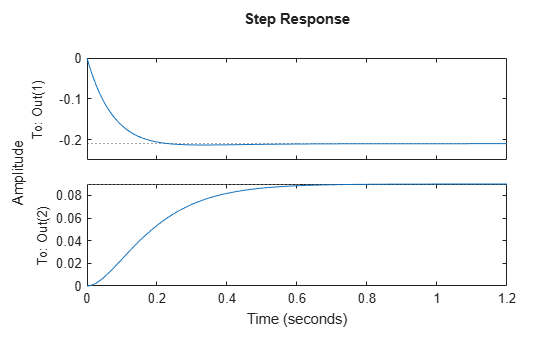## Uncertain State-Space Models

Uncertain state-space (`uss`) models are linear systems with uncertain state-space matrices and/or uncertain linear dynamics. Like their numeric (i.e., not uncertain) counterpart, the `ss` model object, you can build them from state-space matrices using the `ss` command. When one or more of the state-space matrices contain uncertain elements (uncertain Control Design Blocks), the result is a `uss` model object.

Combining uncertain systems with other uncertain systems (for example, using model arithmetic, `connect`, or `feedback`) usually results in an uncertain system. You can also combine numeric systems with uncertain systems. Usually the result is an uncertain system. The nominal value of an uncertain system is a `ss` model object.

In the example below, the `A`, `B` and `C` matrices are made up of uncertain real parameters. Packing them together with the `ss` command results in a continuous-time uncertain system.

### Uncertain State-Space Model

To create an uncertain state-space model, you first use Control Design Blocks to create uncertain elements. Then, use the elements to specify the state-space matrices of the system.

For instance, create three uncertain real parameters and build state-spaces matrices from them.

```p1 = ureal('p1',10,'Percentage',50); p2 = ureal('p2',3,'PlusMinus',[-.5 1.2]); p3 = ureal('p3',0); A = [-p1 p2; 0 -p1]; B = [-p2; p2+p3]; C = [1 0; 1 1-p3]; D = [0; 0];```

The matrices constructed with uncertain parameters, `A`, `B`, and `C`, are uncertain matrix (`umat`) objects. Using them as inputs to `ss` results in a 2-output, 1-input, 2-state uncertain system.

`sys = ss(A,B,C,D)`
```sys = Uncertain continuous-time state-space model with 2 outputs, 1 inputs, 2 states. The model uncertainty consists of the following blocks: p1: Uncertain real, nominal = 10, variability = [-50,50]%, 2 occurrences p2: Uncertain real, nominal = 3, variability = [-0.5,1.2], 2 occurrences p3: Uncertain real, nominal = 0, variability = [-1,1], 2 occurrences Type "sys.NominalValue" to see the nominal value, "get(sys)" to see all properties, and "sys.Uncertainty" to interact with the uncertain elements. ```

The display shows that the system includes the three uncertain parameters.

### Properties of uss Objects

`uss` models, like all model objects, include properties that store dynamics and model metadata. View the properties of an uncertain state-space model.

```p1 = ureal('p1',10,'Percentage',50); p2 = ureal('p2',3,'PlusMinus',[-.5 1.2]); p3 = ureal('p3',0); A = [-p1 p2; 0 -p1]; B = [-p2; p2+p3]; C = [1 0; 1 1-p3]; D = [0; 0]; sys = ss(A,B,C,D); % create uss model get(sys)```
``` NominalValue: [2x1 ss] Uncertainty: [1x1 struct] A: [2x2 umat] B: [2x1 umat] C: [2x2 umat] D: [2x1 double] E: [] StateName: {2x1 cell} StateUnit: {2x1 cell} InternalDelay: [0x1 double] InputDelay: 0 OutputDelay: [2x1 double] Ts: 0 TimeUnit: 'seconds' InputName: {''} InputUnit: {''} InputGroup: [1x1 struct] OutputName: {2x1 cell} OutputUnit: {2x1 cell} OutputGroup: [1x1 struct] Notes: [0x1 string] UserData: [] Name: '' SamplingGrid: [1x1 struct] ```

Most of the properties behave similarly to how they behave for `ss` model objects. The `NominalValue` property is itself an `ss` model object. You can therefore analyze the nominal value as you would any state-space model. For instance, compute the poles and step response of the nominal system.

`pole(sys.NominalValue)`
```ans = 2×1 -10 -10 ```
`step(sys.NominalValue)`As with the uncertain matrices (`umat`), the `Uncertainty` property is a structure containing the uncertain elements. You can use this property for direct access to the uncertain elements. For instance, check the `Range` of the uncertain element named `p2` within `sys`.

`sys.Uncertainty.p2.Range`
```ans = 1×2 2.5000 4.2000 ```

Change the uncertainty range of `p2` within `sys`.

`sys.Uncertainty.p2.Range = [2 4];`

This command changes only the range of the parameter called `p2` in `sys`. It does not change the variable `p2` in the MATLAB workspace.

`p2.Range`
```ans = 1×2 2.5000 4.2000 ```

### Lifting a ss to a uss

A not-uncertain state space object may be interpreted as an uncertain state space object that has no dependence on uncertain elements. Use the `uss` command to “lift” a `ss` to the `uss` class.

```sys = rss(3,2,1); usys = uss(sys) USS: 3 States, 2 Outputs, 1 Input, Continuous System ```

Arrays of `ss` objects can also be lifted. See Array Management for Uncertain Objects for more information about how arrays of uncertain objects are handled.